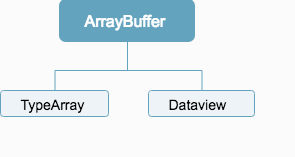VillainHR

## Web 直播流的解析

2017-04-16

Web 进制操作是一个比较底层的话题，因为平常做业务的时候根本用不到太多，或者说，根本用不到。

`老铁，没毛病`

• canvas
• websocket
• file
• fetch
• webgl

## 整体架构

``````let socket = new WebSocket('ws://127.0.0.1:8080');
socket.binaryType = 'arraybuffer';

let arrayBuffer = event.data;
···
});
``````## TypedArray

### 二进制

``````00000000000000000000000100111010
``````

• signed: 从左到右第一位开始，如果为 0 则表示为正，为 1 则表示为负。例如：-127~+127
• unsigned: 从左到右第一位不作为符号的表示。例如：0~255

• 八进制(octet)
• 十进制(Decimal)

### 位运算

Web 中的位运算和其它语言中类似，有基本的 7 个。

#### 与 (`&`)

``````// 在 Web 中二进制不能直接表示
001 & 101 = 001
``````

``````// 和 1000 进行相与
buf & 8
``````

#### 或 (`|`)

``````// FROM MDN
9 (base 10) = 00000000000000000000000000001001 (base 2)
14 (base 10) = 00000000000000000000000000001110 (base 2)
--------------------------------
14 | 9 (base 10) = 00000000000000000000000000001111 (base 2) = 15 (base 10)
``````

#### 非 (`~`)

``````// FROM MDN
9 (base 10) = 00000000000000000000000000001001 (base 2)
--------------------------------
~9 (base 10) = 11111111111111111111111111110110 (base 2) = -10 (base 10)
``````

#### 异或 (`^`)

``````// FROM MDN
9 (base 10) = 00000000000000000000000000001001 (base 2)
14 (base 10) = 00000000000000000000000000001110 (base 2)
--------------------------------
14 ^ 9 (base 10) = 00000000000000000000000000000111 (base 2) = 7 (base 10)
``````

#### 左移 (`<<`)

``````// FROM MDN
9 (base 10): 00000000000000000000000000001001 (base 2)
--------------------------------
9 << 2 (base 10): 00000000000000000000000000100100 (base 2) = 36 (base 10)
``````

#### 带位右移 (`>>`)

``````1001 >> 2 = 1110
``````

#### 直接右移 (`>>>`)

``````1001 >> 2 = 0010
``````

### 优先级

~ >> << >>> & ^ |

### 位运算具体运用

#### 状态改变

• 0： 填完
• 1： 未填
• 2：少填
• 3：填错

• 0001： 填完
• 0010： 未填
• 0100：少填
• 1000：填错

``````var mask = 0001 | 1000;
``````

``````// 是否填错
``````

``````if(mask & (1000 | 0001)) doSth;
``````

``````// 取填完的反状态
var done = ~0001; // 1110

// 添加少填状态;
``````

#### 进制转换

• Number(string): 字面上转换字符串为十进制。

parseInt 用于字符串过滤，例如：

``````parseInt('15px', 10); // return 15
``````

``````parseInt(' 0xF', 16); // return 15
``````

``````Number('0x11')    // 17
Number('0b11')    // 3
Number('0o11')    // 9
``````

toString

toString 里面的坑就没有 parseInt 这么多了。它也是进制转换非常好用的一个工具。因为是 `字符串`，所以，这里就只能针对字面量进制进行转换了–2,8,(10),16。这四种进制的相关之间转换。

``````0b1101101.toString(8); // 155
0b1101101.toString(10); // 109
0b1101101.toString(8); // 6d
``````

``````function hexConvert(str){
return "0x" + str.toString(16);
}
``````

### 整体架构

TypeArray 不是一个可以用程序写出来的概念，它是许多 TypeArray 的总称。参考: TypeArray。可以了解到，它的子类如下：

• Int8Array();
• Uint8Array();
• Uint8ClampedArray();
• Int16Array();
• Uint16Array();
• Int32Array();
• Uint32Array();
• Float32Array();
• Float64Array();

Uint8Array > Uint16Array > Int8Array > …

Int8 1 8位带符号整数 signed char
Uint8 1 8位不带符号整数 unsigned char
Uint8C 1 8位不带符号整数（自动过滤溢出） unsigned char
Int16 2 16位带符号整数 short
Uint16 2 16位不带符号整数 unsigned short
Int32 4 32位带符号整数 int
Uint32 4 32位不带符号的整数 unsigned int
Float32 4 32位浮点数 float
Float64 8 64位浮点数 double

TypeArray 的实例化有 4 种：

``````new TypedArray(length); // 创建指定长度的 typeArray
new TypedArray(typedArray); // 复制新的 typeArray
new TypedArray(object); // 不常用
new TypedArray(buffer [, byteOffset [, length]]); // 参数为 arrayBuffer。
``````

``````var octArr = new Uint8Array(2);
octArr.length;  // 2  用来指代 octArr 元素个数。比如 octArr，octArr
octArr.byteLength; // 2  单位为 B
octArr.BYTES_PER_ELEMENT; // 1 单位为 B

var hexArr = new Uint16Array(2);
hexArr.length; // 2 同上，指代元素个数
hexArr.byteLength; // 4B
hexArr.BYTES_PER_ELEMENT; // 2B
``````

``````// From a length
var uint8 = new Uint8Array(2);
uint8 = 42;
console.log(uint8); // 42
console.log(uint8.length); // 2
console.log(uint8.BYTES_PER_ELEMENT); // 1

// From an array
var arr = new Uint8Array([21,31]);
console.log(arr); // 31

// From another TypedArray
var x = new Uint8Array([21, 31]);
var y = new Uint8Array(x);
console.log(y); // 21

// From an ArrayBuffer
var buffer = new ArrayBuffer(8); // 创建 8个字节长度的 arrayBuffer
var z = new Uint8Array(buffer, 1, 4);
``````

``````var arrBuffer = Uint8Array.from('123'); // [1,2,3]

// 或者

var arrBuffer = Uint8Array.of(1,2,3); // [1,2,3]
``````

#### 多字节图

``````buf; // 10B 的 buf

var firstB = new Uint8Array(buf,0,1); // buf 中第一个字节内容

var theRestB = new Uint8Array(buf,1,9); // buf 中 2~10 的字节内容
``````

#### 字节概念

Uint8 每一个数组位，表示 8 位二进制，即范围为 0~255。

``````var arrBuffer = Uint8Array.from('61545');
arrBuffer; // [6, 1, 5, 4, 5]
``````

``````arrBuffer += 1; // 7

arrBuffer += 0xfe; // 6。因为 7 + 254 溢出 6
``````

• 大字节序：假如 0xAABB 被 Uint16 存储为 2 位。那么按照大字节序就是按顺序来，即 0: 0xAA, 1:0xBB。
• 小字节序：和上面相反，即，0:0xBB,1:0xAA。

``````(function () {
let buf = new ArrayBuffer(2);
(new DataView(buf)).setInt16(0, 256, true);  // little-endian write
return (new Int16Array(buf)) === 256;  // platform-spec read, if equal then LE
})();
``````

## DataView

DataView 没有 `TypeArray` 这么复杂，衍生出这么多个 Uint/IntArray。它就是一个构造函数。同样，它的目的也是对底层的 arrayBuffer 进行读取。那么，为什么它会被创建出来呢？

``````new DataView(buffer [, byteOffset [, byteLength]])
``````

• buffer: 需要接入的底层 ArrayBuffer
• byteOffset: 偏移量，单位为字节
• byteLength: 获取长度，单位为字节

``````dataview.getInt16(byteOffset [, littleEndian]);
// 根据字节序，获得偏移字节后的两个字节。
``````
• byteOffset: 单位为 字节。
• littleEndian[boolean]: 字节序。默认为 false。表示大字节序。
``````var buffer = new ArrayBuffer(8);
var dataview = new DataView(buffer);
dataview.getInt16(1,true); // 0
``````

## Buffer 场景

• canvas
• websocket
• file
• fetch
• webgl

### file

``````let fileInput = document.getElementById('fileInput');
let file = fileInput.files;
···
};
``````

### AJAX

``````let xhr = new XMLHttpRequest();
xhr.open('GET', someUrl);
xhr.responseType = 'arraybuffer';

let arrayBuffer = xhr.response;
···
};

xhr.send();
``````

### fetch

``````fetch(url)
.then(request => request.arrayBuffer())
.then(arrayBuffer => ···);
``````

### canvas

``````let canvas = document.getElementById('my_canvas');
let context = canvas.getContext('2d');
let imageData = context.getImageData(0, 0, canvas.width, canvas.height);
let uint8ClampedArray = imageData.data;
``````

### websocket

``````let socket = new WebSocket('ws://127.0.0.1:8080');
socket.binaryType = 'arraybuffer';